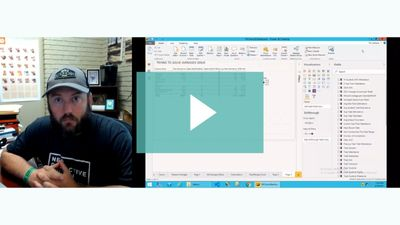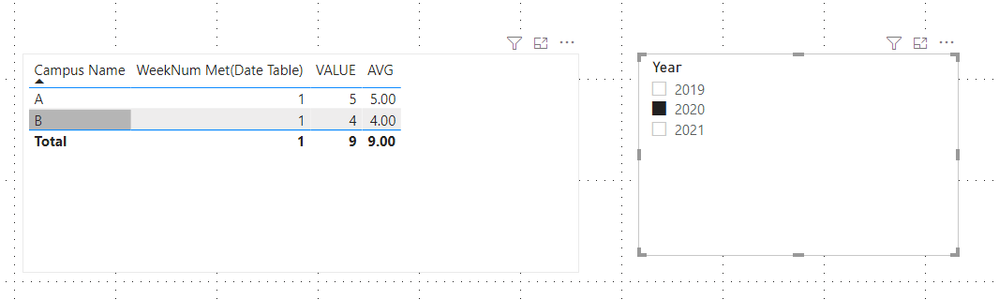cancel
Showing results for
Did you mean:Regular Visitor

## Trouble with getting averages for filtered time period and filtered location

Hi everyone. I'm new to the community and this is my first post. I recorded a video of me walking through my issue. If anyone has any ideas on how to help, let me know.Soapbox - Trouble figuring out averages

1 ACCEPTED SOLUTIONCommunity Support

Hi @timlem

From your video, I know your problem is that when you distinctcount weeknum from Date table, you will always get whole weeknums 52 instead of correct result from Fact table. You can try to build a measure to get correct result.

My Sample:Sometimes we will get wrong result from weeknum function at the end of the previous year and the beginning of the next year.

For example:

In my Sample I let week start on Sunday.

2020/12/31,2021/01/01,2021/01/02 are in the same weeknum, but weeknum function will give you 53 in 2020 and 1 in 2021. It's wrong result.

``````Date =
CALENDAR ( DATE ( 2019, 01, 01 ), DATE ( 2021, 12, 31 ) ),
"Year", YEAR ( [Date] ),
"Month", MONTH ( [Date] ),
"WeekNum", WEEKNUM ( [Date], 1 ),
"WeekDay", WEEKDAY ( [Date], 1 ),
"MonthName", FORMAT ( [Date], "MMMM" ),
"DayName", FORMAT ( [Date], "DDDD" )
)``````

``````ISO 8601 WeekNum =
VAR _COUNT0 =
CALCULATE (
COUNTROWS ( 'Date' ),
FILTER (
'Date',
'Date'[Year] = EARLIER ( 'Date'[Year] )
&& 'Date'[WeekNum] - 1 = 0
)
)
VAR _BASENUM1 =
IF ( _COUNT0 < 7, 'Date'[WeekNum] - 1, 'Date'[WeekNum] )
VAR _ISO_8601_WeekNum =
IF (
WEEKDAY ( DATE ( 'Date'[Year] - 1, 01, 01 ) ) <> 1
&& 'Date'[Year] = 'Date'[Year]
&& _BASENUM1 = 0,
WEEKNUM ( DATE ( MIN ( 'Date'[Year] ), 12, 31 ), 1 ) - 1,
_BASENUM1
)
RETURN
_ISO_8601_WeekNum``````
``````ISO WeekYear =
VAR _COUNT0 =
CALCULATE (
COUNTROWS ( 'Date' ),
FILTER (
'Date',
'Date'[Year] = EARLIER ( 'Date'[Year] )
&& 'Date'[WeekNum] - 1 = 0
)
)
VAR _BASENUM1 =
IF ( _COUNT0 < 7, 'Date'[WeekNum] - 1, 'Date'[WeekNum] )
RETURN
IF(_BASENUM1 = 0,'Date'[Year] -1,'Date'[Year])``````

Measure:

``````WeekNum Met(Date Table) =
VAR _T =
'Fact',
"YearWeek",
RELATED ( 'Date'[ISO WeekYear] ) * 100
+ RELATED ( 'Date'[ISO 8601 WeekNum] )
)
VAR _T2 =
SUMMARIZE ( _T, [YearWeek] )
RETURN
COUNTAX ( _T2, [YearWeek] )``````

Result is as below.Best Regards,
Rico Zhou

If this post helps, then please consider Accept it as the solution to help the other members find it more quickly.

3 REPLIES 3Community Support

Hi @timlem

Could you tell me if your problem has been solved? If it is, kindly Accept it as the solution. More people will benefit from it. Or you are still confused about it, please provide me with more details about your table and your problem or share me with your pbix file from your Onedrive for Business.

Best Regards,

Rico ZhouRegular Visitor

I would also be up for hopping on zoom to troubleshoot together.Community Support

Hi @timlem

From your video, I know your problem is that when you distinctcount weeknum from Date table, you will always get whole weeknums 52 instead of correct result from Fact table. You can try to build a measure to get correct result.

My Sample:Sometimes we will get wrong result from weeknum function at the end of the previous year and the beginning of the next year.

For example:

In my Sample I let week start on Sunday.

2020/12/31,2021/01/01,2021/01/02 are in the same weeknum, but weeknum function will give you 53 in 2020 and 1 in 2021. It's wrong result.

``````Date =
CALENDAR ( DATE ( 2019, 01, 01 ), DATE ( 2021, 12, 31 ) ),
"Year", YEAR ( [Date] ),
"Month", MONTH ( [Date] ),
"WeekNum", WEEKNUM ( [Date], 1 ),
"WeekDay", WEEKDAY ( [Date], 1 ),
"MonthName", FORMAT ( [Date], "MMMM" ),
"DayName", FORMAT ( [Date], "DDDD" )
)``````

``````ISO 8601 WeekNum =
VAR _COUNT0 =
CALCULATE (
COUNTROWS ( 'Date' ),
FILTER (
'Date',
'Date'[Year] = EARLIER ( 'Date'[Year] )
&& 'Date'[WeekNum] - 1 = 0
)
)
VAR _BASENUM1 =
IF ( _COUNT0 < 7, 'Date'[WeekNum] - 1, 'Date'[WeekNum] )
VAR _ISO_8601_WeekNum =
IF (
WEEKDAY ( DATE ( 'Date'[Year] - 1, 01, 01 ) ) <> 1
&& 'Date'[Year] = 'Date'[Year]
&& _BASENUM1 = 0,
WEEKNUM ( DATE ( MIN ( 'Date'[Year] ), 12, 31 ), 1 ) - 1,
_BASENUM1
)
RETURN
_ISO_8601_WeekNum``````
``````ISO WeekYear =
VAR _COUNT0 =
CALCULATE (
COUNTROWS ( 'Date' ),
FILTER (
'Date',
'Date'[Year] = EARLIER ( 'Date'[Year] )
&& 'Date'[WeekNum] - 1 = 0
)
)
VAR _BASENUM1 =
IF ( _COUNT0 < 7, 'Date'[WeekNum] - 1, 'Date'[WeekNum] )
RETURN
IF(_BASENUM1 = 0,'Date'[Year] -1,'Date'[Year])``````

Measure:

``````WeekNum Met(Date Table) =
VAR _T =
'Fact',
"YearWeek",
RELATED ( 'Date'[ISO WeekYear] ) * 100
+ RELATED ( 'Date'[ISO 8601 WeekNum] )
)
VAR _T2 =
SUMMARIZE ( _T, [YearWeek] )
RETURN
COUNTAX ( _T2, [YearWeek] )``````

Result is as below.Best Regards,
Rico Zhou

If this post helps, then please consider Accept it as the solution to help the other members find it more quickly.